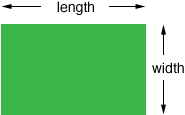Quandaries and Queries Who is asking: Parent Level: Elementary Question: My stepdaughter is in 5th grade and they are preparing for the state standard tests. Her teacher has been giving them worksheets with word problems that will be types of questions on the test. The following is a question that my husband and I are having trouble helping her with, PLEASE HELP! Nate's garden has a perimeter of 34 meters and an area of 72 square meters. What are the dimensions of the garden? Hi Rebecca,If the perimeter is 34 meters then half way around the garden is 34/2 = 17 meters. Thus length + width = 17 meters. The area is 72 square meters so lengthwidth = 72 square meters. As your daughter if she knows two numbers which have a product of 72. Is their sum 17? If not find a different pair of numbers that have a product of 72. Is their sum 17? If not, try again. Penny Go to Math Central